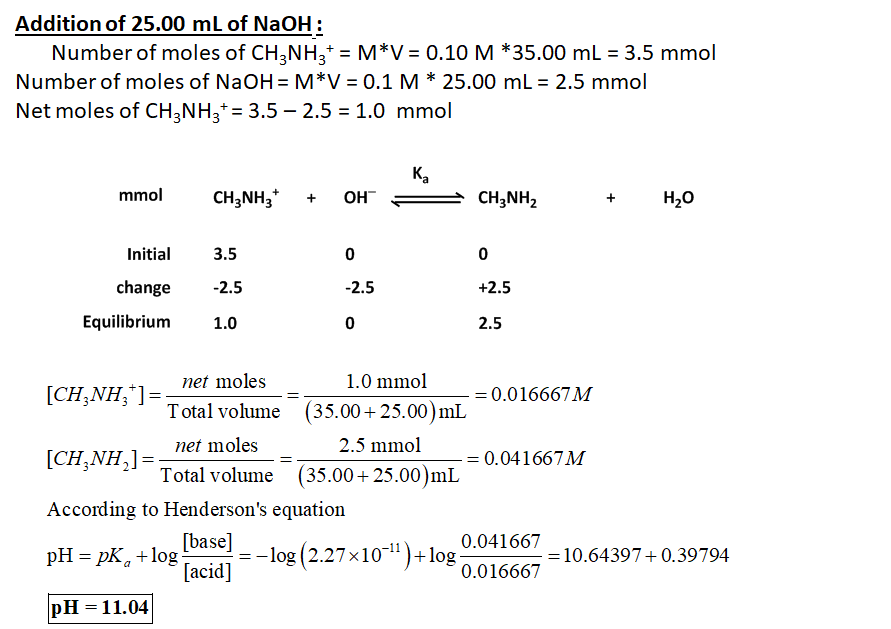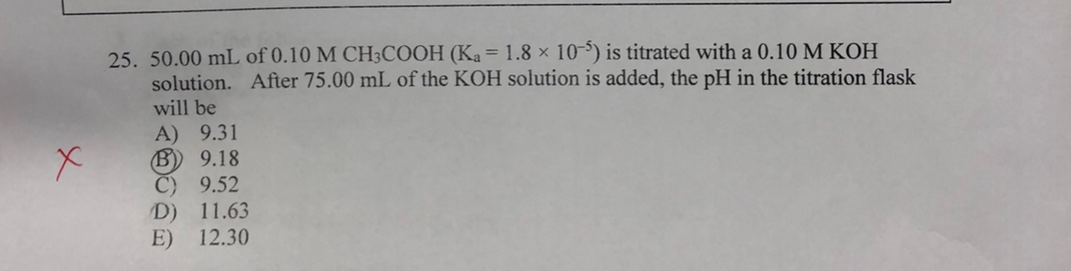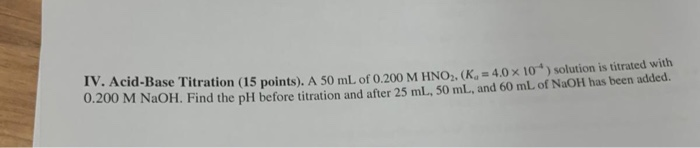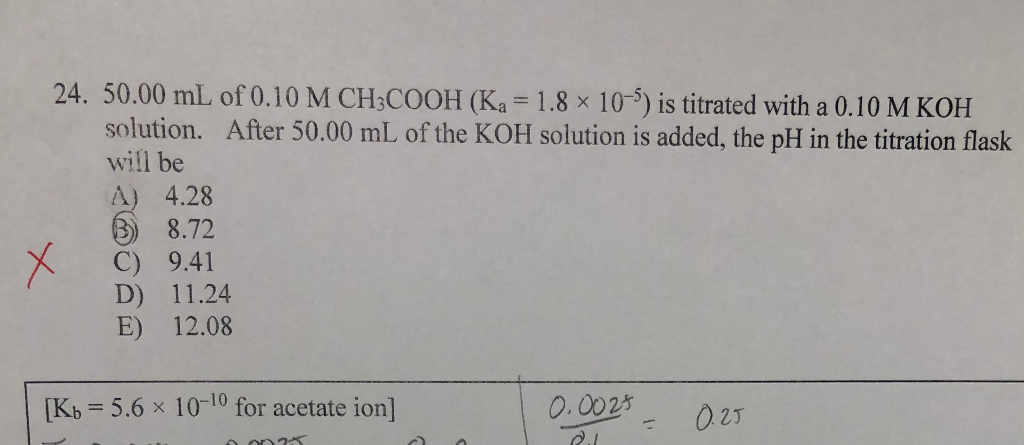Question

# A chemist performs a titration experiment on a 35 mL sample of 0.10 M CH3NH3+ (Ka...

A chemist performs a titration experiment on a 35 mL sample of 0.10 M CH3NH3+ (Ka = 2.27 x 10−11) with 0.10 M NaOH. Determine the pH of solution after 25 mL of NaOH is added to the solution.#### Earn Coins

Coins can be redeemed for fabulous gifts.

Similar Homework Help Questions
• ### A titration is performed on a 25 mL sample of 0.15 M HCN (Ka = 4.9...

A titration is performed on a 25 mL sample of 0.15 M HCN (Ka = 4.9 x 10–10) with 0.30 M NaOH. Which of the following is the pH of the solution after 15 mL of NaOH is added?

• ### 25. 50.00 mL of 0.10 M CH3COOH (Ka = 1.8 x 10") is titrated with a...25. 50.00 mL of 0.10 M CH3COOH (Ka = 1.8 x 10") is titrated with a 0.10 M KOH solution. After 75.00 mL of the KOH solution is added, the pH in the titration flask will be A) 9.31 B) 9.18 C) 9.52 D) 11.63 E) 12.30

• ### Consider a titration of 250 mL 0.15 M acetic acid (Ka = 1.8 x10-5) with 0.10...

Consider a titration of 250 mL 0.15 M acetic acid (Ka = 1.8 x10-5) with 0.10 M KOH. What is the pH of the acetic acid solution (ie: before the titration has begun?) What is the pH after adding 25 mL of 0.10 M KOH? What is the volume of base needed to reach the equivalence point? Is the pH at equivalence point acidic, basic, or exactly neutral? What is the pH after 500 mL of KOH has been added?

• ### A student performs a titration of 50.0 mL of 0.100 M hydrofluoric acid (HF), using0.050 M...

A student performs a titration of 50.0 mL of 0.100 M hydrofluoric acid (HF), using0.050 M sodium hydroxide (NaOH). The Ka for hydrofluoric acid is 8.8 x 10-5 What is the pH of the solution after the addition of 20.0 mL of sodium hydroxide solution?

• ### IV. Acid-Base Titration (15 points). A 50 mL of 0.200 M HNO2, (Ka= 4.0 x 10)...IV. Acid-Base Titration (15 points). A 50 mL of 0.200 M HNO2, (Ka= 4.0 x 10) solution is titrated with 0.200 M NaOH. Find the pH before titration and after 25 mL, 50 mL, and 60 mL of NaOH has been added.

• ### Consider the titration of 50 mL of 0.100 M acetic acid (CH3COOH, Ka = 1.8 x...

Consider the titration of 50 mL of 0.100 M acetic acid (CH3COOH, Ka = 1.8 x 10-5) with 0.200 M NaOH solution. Show all calculations for full credit. a) Write the titration reaction: b) Calculate the pH after 5.00 mL of NaOH: c) Calculate the pH after 12.5 mL of NaOH: d) Calculate the pH after 25 mL of NaOH:

• ### In the titration of 25.00 ml of 0.10 M HF with 0.10 M NaOHn given ka...

In the titration of 25.00 ml of 0.10 M HF with 0.10 M NaOHn given ka = 3.5 x 10-4 find initial PH, PH at the equivalence point and Ph at half equivalence point

• ### 24. 50.00 mL of 0.10 M CH3COOH (Ka = 1.8 x 10-5) is titrated with a 0.10 M KOH solution. After 50.00 mL of the KOH solu...24. 50.00 mL of 0.10 M CH3COOH (Ka = 1.8 x 10-5) is titrated with a 0.10 M KOH solution. After 50.00 mL of the KOH solution is added, the pH in the titration flask will be A) 4.28 B) 8.72 C) 9.41 D) 11.24 E) 12.08 [Kb = 5.6 x 10-10 for acetate ion] 0.0025 - 0.25 .

• ### During the titration of 50.00 mL of 0.202 M weak acid solution, Ka = 3.76 x...

During the titration of 50.00 mL of 0.202 M weak acid solution, Ka = 3.76 x 10-7, what is the pH after 25.00 mL of 3.76 M NaOH solution has been added?

• ### 25 mL of 0.10 M acetic acid is titrated with 0.10 M NaOH. What is the...

25 mL of 0.10 M acetic acid is titrated with 0.10 M NaOH. What is the pH after 30 ml of NaOH have been added? Ka for acetic acid = 1.8 x 10^-5.